# Constructors in C++

## Constructors in C++ with Examples

In this article, I am going to discuss Constructors in C++ with Examples. Please read our previous article, where we discussed Data Hiding in C++ with Examples. At the end of this article, you will understand what are Constructors and their type as well as their role and responsibility in C++ Applications with Examples.

##### Why do we need Constructors in C++?

Before understanding what are constructors, let’s first understand why we need Constructors in C++. For a better understanding please have a look at the below code.

```class Rectangle
{
private:
int length;
public:
void setLength (int l)
{
if (l >= 0)
length = l;
else
length = 0;
}
{
if (b >= 0)
else
}
int getLength ()
{
return length;
}
{
}
int Area ()
{
}
int Perimeter ()
{
return 2 * (length + breadth);
}
};
```

Here we have a class called Rectangle with length and breadth as data members as well as the accessor and mutator methods for these two data members. Now we will create an object class Rectangle.

Rectangle r;

The object of the Rectangle class is created having length and breadth as shown in the below image.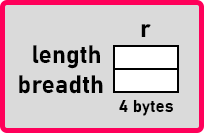Now, we can access all the functions of class Rectangle through object r. Now the problem is that we have created an object of Rectangle. We got length and breadth. But what are the values stored in them? There are no values. Did we initialize them? No. So they have garbage values. Then we want to set the length. So, we should call the setLength and setBreadth functions. We are calling these functions which will take the given value and set them to length and breadth data members. This Philosophically is totally wrong. Let’s see how.

We have created an object of Rectangle. Later we set the length and breadth data members. There is a problem. What is that? Suppose we have purchased a rectangle from the market. So, when we purchase it, was it not have any length and breadth? When we buy something from the market or when we get something constructed in some factory and when they hand over the thing to us then that thing will have some properties. Suppose if it is a rectangle then it must have some length and breadth. How it can be garbage random length and breadth? There must be some length and breadth, not just garbage.

Let’s understand the same concept with one more example. Suppose we have a car and color is the property of the car.

You have bought a car and the car will have some color. What is the color of the car? No color. How it is possible that you bought a car from the market and it has no color. When you placed an order at that time only you said that I want a white color car or any other color car. So, the color of this car is white. It is not like first you will buy a car and bring it home and then you give white color.

Coming back to our example, first, we are creating a rectangle object, and then only we are setting its length and breadth data members. This Philosophically is wrong. When you booked the car, at that time only you said that the car color should be white and then you should get a white car. That’s it. So, when the car is delivered, it is having some color, not garbage.

In the same way, when you are creating an object of Rectangle at that time only, we should be able to say that I want the length of 10 and a breadth of 5. You don’t have to set it up later. So, this is the philosophy behind that one.

We want the length and breadth to be set at the time of construction of that object. So, what we should have inside the class? What is visible or publicly accessible? Functions. So, we should have a function that should automatically be called when an object is constructed. That function will take values of length and breadth as parameters and assign those values. These functions are known as Constructors in C++.

##### What are Constructors in C++?

C++ allows the object to initialize itself when it is created. This automatic initialization of the object is known as Constructors. The constructor in C++ is a block of code that initializes the newly created object.

A constructor initializes an object immediately upon creation. It has the same name as the class in which it resides and is syntactically similar to a method. Once defined, the constructor is called automatically immediately while creating the object. Constructors have no return type, not even void.

So, in simple words, we can define the constructors in C++ are the special types of methods of a class that are automatically executed whenever we create an instance (object) of that class. The Constructors are responsible for two things. One is the object initialization and the other one is memory allocation.

##### Constructors Examples in C++:

Now, let us try to understand constructors with some examples. Please have a look at the below class. The following is a class with the name Rectangle having two private data members called length and breadth.

```class Rectangle {
private:
int length;
};
```

Now, let us create an object of the Rectangle class as follows:

Rectangle r;

An object of Rectangle is created and it will be shown in the memory as shown in the below image.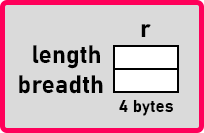As you can see in the above image, this object (r) is having length and breadth data members. We can call it as the object is constructed. Who constructed it? There is a function that constructed it i.e. constructor. Did we write any function in the class? No. Then who wrote the function? The compiler will provide a function for this when it converts the class code into machine code. That is not visible to us. That constructor will create this object. That means every class will have some constructor. If we don’t provide any constructor explicitly, then the compiler provides a built-in constructor which is called as default constructor.

##### Types of Constructors in C++:

A constructor is a function that will have the same name as the class name. Can we write our own constructor? Yes, we can write our own constructors. Then how we can write? We can write three different types of constructors.

1. Non-Parameterized Constructor
2. Parameterized Constructor
3. Copy Constructor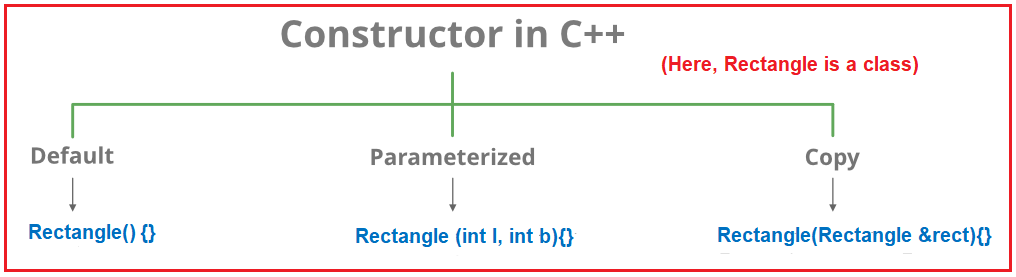Note: If you don’t write any of them then a default constructor is provided by the compiler. Sometimes the non-parameterized constructor is also called a default constructor and the default is called also called a built-in constructor. Now, let us understand all these constructors through examples.

##### Default Constructor in C#:

The Constructor without a parameter is called the default constructor in C++. Again, the default constructor in C++ is classified into two types. They are as follows.

1. System-Defined Default Constructor / Built-in Constructor
2. User-Defined Default Constructor
##### What is System Defined Default Constructor in C#?

If we are not defined any constructor explicitly in our class, then by default the compiler will provide one constructor while generating the machine code. That constructor is called a default constructor. The default constructor will assign default values to the data members. As this constructor is created by the system this is also called a system-defined default constructor.

##### Example to Understand Default Constructor in C++:

In the below example, within the Rectangle class, we have not defined any constructor explicitly. So, while generating the machine code, the compiler will provide the default constructor.

```#include <iostream>
using namespace std;
class Rectangle
{
private:
int length;
public:

void Display()
{
cout << "Length: " << length << " Breadth: " << breadth;
}
};

int main()
{
Rectangle r;
r.Display();
}
```
###### Output: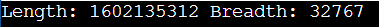Note: The point that you need to remember is that the compiler will only provide the default constructor if as a programmer you are not defined any constructor explicitly in our class.

##### When do we need to provide the constructor explicitly in C++?

If you want to execute some logic at the time of object creation, that logic may be object initialization logic or some other useful logic, then as a developer, we should define the constructor explicitly in our class.

##### What is a User-Defined Default Constructor in C++?

The constructor which is defined by the user without any parameter is called a user-defined default constructor in C++. This constructor does not accept any argument but as part of the constructor body, you can write your own logic. This is also called a non-parameterized constructor as it does not take any parameter. The following is the syntax.

Rectangle()
{
length = 10;
}

##### Example of User-Defined Default Constructor in C++:

In the below example, we gave created the constructor without a parameter. This is called a user-defined default constructor. As part of the constructor body, we have initialized the length and breadth data members with the values 10 and 20 respectively. So, when we created an object of the Rectangle class, this constructor will call automatically and set the length and breadth values 10 and 20.

```#include <iostream>
using namespace std;
class Rectangle
{
private:
int length;
public:
Rectangle ()
{
length = 10;
}

void Display()
{
cout << "Length: " << length << " Breadth: " << breadth;
}
};

int main()
{
Rectangle r;
r.Display();
}
```
###### Output: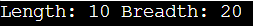The drawback of the above user-defined default constructor is that every instance (i.e. for every object) of the class will be initialized (assigned) with the same values. That means it is not possible to initialize each instance of the class with different values.

##### When should we define a Parameterized Constructor in a Class?

If we want to initialize the object dynamically with the user-given values then we need to use the parameterized constructor in C++. The advantage is that we can initialize each object with different values.

##### What is Parameterized Constructor in C++?

The user-given constructor with parameters is called the parameterized constructor in C++. With the help of a Parameterized constructor, we can initialize each instance of the class with different values. That means using parameterized constructor we can store a different set of values in different objects created in the class. Following is the syntax.

Rectangle (int l, int b){
length = l;
}
The following is the syntax to create the Rectangle object.
Rectangle r1(10, 20);
Rectangle r2(30, 40);

##### Example to understand the Parameterized Constructor in C++:

In the below example, we have created the constructor which takes two integer parameters and then sets the values of length and breadth data members respectively. Now, while creating the Rectangle object, we need to provide the values for the l and b parameters of the constructor.

```#include <iostream>
using namespace std;
class Rectangle
{
private:
int length;
public:
Rectangle (int l, int b){
length = l;
}

void Display()
{
cout << "Length: " << length << " Breadth: " << breadth;
}
};

int main()
{
Rectangle r1(10, 20);
r1.Display();
cout << endl;
Rectangle r2(30, 40);
r1.Display();
}
```
###### Output: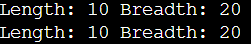##### How many constructors can be defined in a class in C++?

In C++, in a class, we can define one no-argument constructor plus “n” number of parameterized constructors. But the most important point that you need to remember is that each and every constructor must have a different signature. A different signature means the number, type, and parameter order should be different.

##### What is Copy Constructor in C++?

The constructor which takes a parameter of the class type is called a copy constructor. This constructor is used to copy one object’s data into another object. The main purpose of the copy constructor is to initialize a new object (instance) with the values of an existing object (instance). The following is the syntax to create a Copy Constructor in C++

Rectangle(Rectangle &rect){
length = rect.length;
}

This is a copy constructor. Here, usually, we take objects by reference and not by value. So that when we are calling a constructor, a new rectangle object should not be created. So that’s why we take it as a reference. Then inside the constructor, we assign length and breadth to the length and breadth of the rect object. Following is the syntax to call the Copy Constructor in C++.

Rectangle r2(r1);

We have sent the r1 object as a parameter and this will create another rectangle object that is r2. So r will become “rect” here.

##### Example to understand the Copy Constructor in C++

In the below example, we have created two constructors. One is the parameterized constructor which takes two integer parameters. The second one is also a parameterized constructor which takes the class reference as a parameter as it takes the class reference is a parameter, so it is also called a copy constructor.

```#include <iostream>
using namespace std;
class Rectangle
{
private:
int length;
public:
Rectangle (int l, int b){
length = l;
}

Rectangle(Rectangle &rect){
length = rect.length;
}

void Display()
{
cout << "Length: " << length << " Breadth: " << breadth;
}
};

int main()
{
Rectangle r1(10, 20);
r1.Display();
cout << endl;
Rectangle r2(r1);
r2.Display();
}
```
###### Output: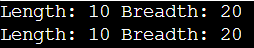##### What is the Philosophy Behind the Constructor?

Now I will explain to you what is the philosophy behind the constructor. Let us understand where we use non-parameterized, parameterized, and copy constructors.

Suppose you have placed an order for manufacturing a car or often we directly go to the market and buy the items. That item was also manufactured already. They will keep it ready-made. Just go and buy whatever you want.

Now let us take another example. Suppose you have gone to a shop to buy a marker. Now, what are the options you have to buy a marker? First, you go to a shop and say “give me a marker”. You did not specify a brand name and a color for the marker. You did not mention anything just said I want a marker.

When you said just, I want a marker, whatever the frequently sold marker is there in the market or in the shop, he will simply hand over that marker to you. When you said just pen, so commonly use the blue color pen of commonly running brand. So, you will get that pen. So, you did not give the color or brand so we can say it is non-parameterized. This is the first method.

I have a rectangle. Initially, length and breadth are 0 later we can change it but initially, there should be something. It cannot be garbage. Then the second method is when you go to a shop and say “I want a red color marker of “xyz” brand”. So, you are mentioning some details here and he will give you that marker only. So, you have given the parameters. We can say it is parameterized.

We want a rectangle of length should be 10 and a breadth should be 5. The third method is when you went to a shop and you are showing him a marker and you want a marker like you to have or copy of that one. So, he will give a new marker for you that is a copy of the marker that you already have.

Now we can understand there are three methods of buying things from a market.

1. The first one is just going out and saying I want this. He will give you. If you go to a restaurant or food store and say “I want pizza”. If the seller has only one category of pizza or commonly people take the same type of pizza, they will hand it over to you.
2. Second, if I want a particular type of pizza like cheese, tomato, Sause, and so on then this is known as parameterized as I am specifying the pizza type.
3. The third is if any person has ‘x’ type of pizza then I will say that I want that ‘x’ type of pizza that is a copy of ‘x’.
##### Points to Remember while working with Constructors in C++:
1. A constructor is a member function of a class
2. It will have the same name as the class name
3. It will not have a return type
4. It should be public
5. It can be declared private also in some cases
6. It is called when the object is created
7. It is used for initializing an object
9. If it is not defined then the class will have a default constructor
10. Constructors can take default arguments
##### Types of Constructors:
1. Non-argument constructor
2. Parameterized constructor
3. Copy constructor
##### All types of Member Functions:
1. Constructors – called when the object is created
2. Accessors – used for knowing the value of data members
3. Mutators – used for changing the value of data member
4. Facilitator – actual functions of class
5. Enquiry – used for checking if an object satisfies some condition
6. Destructor – used for releasing resources used by the object

So, this is the purpose of the constructor and usage of the constructor. Now let us write the complete program for constructors

##### Example to Understand Constructors in C++:
```#include <iostream>
using namespace std;
class Rectangle
{
private:
int length;
public:
Rectangle (int l = 0, int b = 0)
{
setLength (l);
}
Rectangle (Rectangle & rect)
{
length = rect.length;
}
void setLength (int l)
{
if (l >= 0)
length = l;
else
length = 0;
}
{
if (b >= 0)
else
}
int getLength ()
{
return length;
}
{
}
};

int main()
{
Rectangle r;
Rectangle r1 (3);
Rectangle r2 (4, 7);
cout << "r:" << endl;
cout << "Length: " << r.getLength () << " Breadth: " << r.
cout << "r1:" << endl;
cout << "Length: " << r1.getLength () << " Breadth: " << r1.
cout << "r2:" << endl;
cout << "Length: " << r2.getLength () << " Breadth: " << r2.
}
```
###### Output: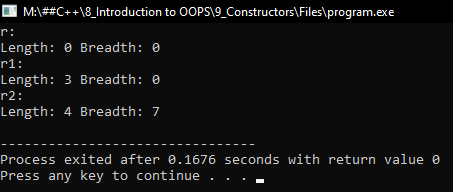##### How constructors are different from a normal member function?

A constructor is different from normal functions in the following ways:

1. The Constructor has the same name as the class name itself.
2. Default Constructors don’t have input arguments however, Copy and Parameterized Constructors can have input arguments
3. A Constructor should not have a return type, even not void.
4. The constructor will automatically call when an object is created.
5. It must be placed in the public section of the class.
6. If we do not specify a constructor, the C++ compiler generates a default constructor for the object.
##### Difference Between Functions and Constructors in C++
###### Constructors
1. It is a special type of function used to initialize objects of their class.
2. The purpose of a constructor is to create an object of a class.
3. Constructor is called automatically when we are creating an object.
4. They are used to initialize objects that don’t exist.
5. The name of the constructor should be the same as the class name of the class.
6. They are not inherited by subclasses.
###### Functions:
1. Functions are a set of instructions that are invoked at any point in a program to perform a specific task.
2. The purpose of a function is to execute C++ code to perform a specific task.
3. We need to call explicitly a function to execute.
4. They perform operations on already created objects.
5. We can give any valid name to functions in C++.
6. They are inherited by subclasses.

1.